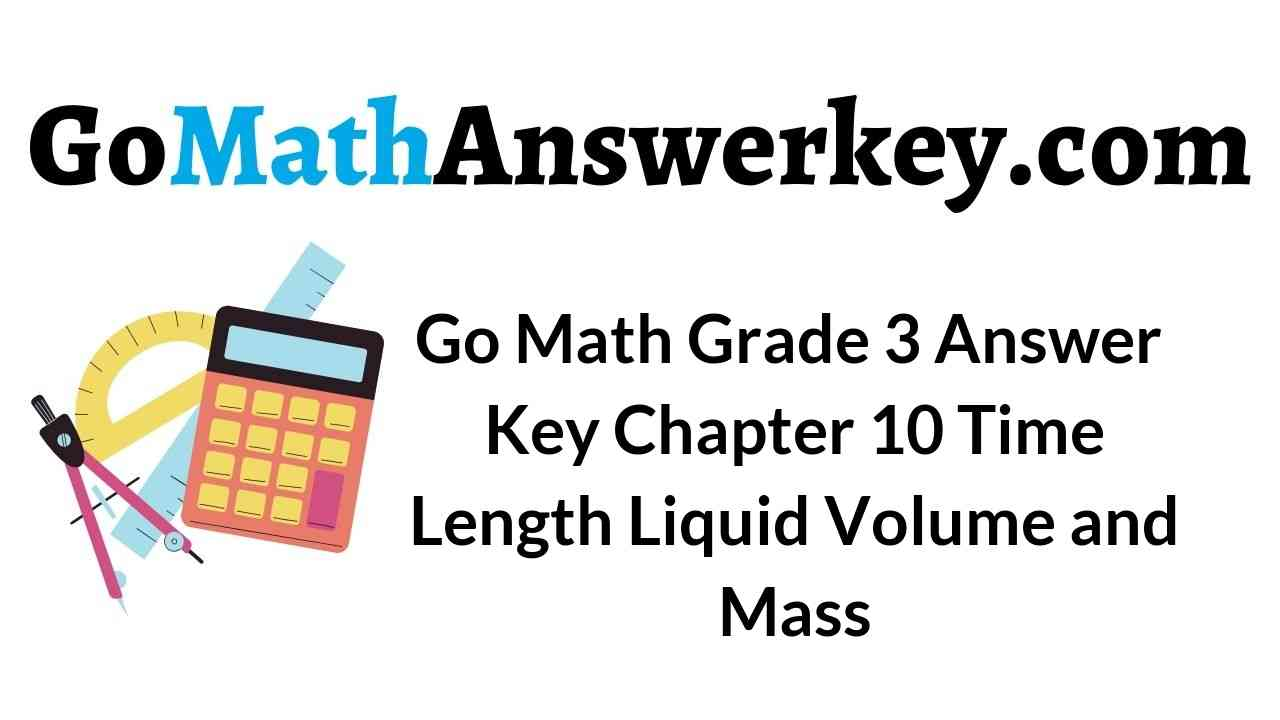# Go Math Grade 3 Chapter 10 Answer Key Pdf Time, Length, Liquid Volume, and Mass

### Time to the Minute – Page No. 565

Write the time. Write one way you can read the time.

Question 1.Answer:  1:16; sixteen minutes after one

Explanation:
Count on by fives and ones from the 12 on the clock to where the minute hand is pointing. Write the missing counting numbers next to the clock.
Write: 1 Hour 16 minutes

Question 2.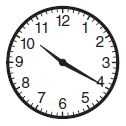Type below:
___________

Answer: 10:20; twenty minutes after ten

Explanation:
Count by fives and ones from the 12 on the clock to where the minute hand is pointing. Write the missing counting numbers next to the clock.
Write: 10 Hours 20 minutes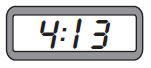Type below:
____________

Answer: 4:13;  thirteen minutes after four

Explanation:
Count by fives and ones from the 12 on the clock to where the minute hand is pointing. Write the missing counting numbers next to the clock.
Write: 4 Hour 13 minutes

Question 4.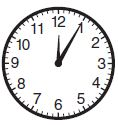Type below:
____________

Answer: 12:05; five minutes after twelve

Explanation:
Count by fives and ones from the 12 on the clock to where the minute hand is pointing. Write the missing counting numbers next to the clock.
Write: 12 Hour 05 minutes

Question 5.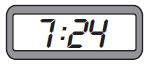Type below:
____________

Answer: 7:24; twenty-four minutes after seven

Explanation:
Count on by fives and ones from the 12 on the clock to where the minute hand is pointing. Write the missing counting numbers next to the clock.
Write: 7 Hour 24 minutes

Question 6.Type below:
____________

Answer: 2:51; nine minutes before three

Explanation:
Count by fives and ones from the 12 on the clock back to where the minute hand is pointing. Write the missing counting numbers next to the clock.
Write: 2:51
nine minutes before three

Write the time another way.

Question 7.
23 minutes after 4
______ : ______

Explanation:
Count on by fives and ones from the 12 on the clock to where the minute hand is pointing. Write the missing counting numbers next to the clock.

Question 8.
18 minutes before 11
______ : ______

Explanation:
Count by fives and ones from the 12 on the clock back to where the minute hand is pointing. Write the missing counting numbers next to the clock.

Question 9.
10 minutes before 9
______ : ______

Explanation:
Count by fives and ones from the 12 on the clock back to where the minute hand is pointing. Write the missing counting numbers next to the clock.

Question 10.
7 minutes after 1
______ : ______

Explanation:
Count on by fives and ones from the 12 on the clock to where the minute hand is pointing. Write the missing counting numbers next to the clock.

Problem Solving

Question 11.
What time is it when the hour hand is a little past the 3 and the minute hand is pointing to the 3?
______ : ______

Explanation:
Count on by fives and ones from the 12 on the clock to where the minute hand is pointing. Write the missing counting numbers next to the clock.

Question 8.

Question 12.
Pete began practicing at twenty-five minutes before eight. What is another way to write this time?
______ : ______

Explanation:
Count by fives and ones from the 12 on the clock back to where the minute hand is pointing. Write the missing counting numbers next to the clock.

### Time to the Minute – Page No. 566

Lesson Check

Question 1.
Which is another way to write 13 minutes before 10?
Options:
a. 9:47
b. 10:13
c. 10:47
d. 11:13

Explanation:
Count by fives and ones from the 12 on the clock back to where the minute hand is pointing. Write the missing counting numbers next to the clock.

Question 2.
What time does the clock show?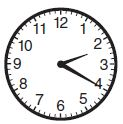Options:
a. 2:20
b. 2:40
c. 3:20
d. 4:10

Explanation:
Count on by fives and ones from the 12 on the clock to where the minute hand is pointing. Write the missing counting numbers next to the clock.

Spiral Review

Question 3.
Each bird has 2 wings. How many wings will 5 birds have?
Options:
a. 7
b. 8
c. 9
d. 10

Explanation:
STEP 1 Draw 2 counters in each group.
STEP 2 Skip count to find how many wings in all. Skip count by 2s until you say 5 numbers (groups)
There are 5 groups with 2 wings in each group. So, there are 10 wings in all.

Find the unknown factor.
9 × ■ = 36
Options:
a. 4
b. 6
c. 8
d. 27

Explanation:
Count how many rows of 36 counters there are.
There are  9 rows of 36 counters. The unknown factor is 4 (c0lumns). n = 4
9× 4 = 36

Question 5.
Mr. Wren has 56 paintbrushes. He places 8 paintbrushes on each of the tables in the art room. How many tables are in the art room?
Options:
a. 6
b. 7
c. 9
d. 48

Explanation:
STEP 1 Draw 56 counters.
STEP 2 Make a group of 8 counters by drawing a circle around them. Continue circling groups of 8 until all 56 counters are in groups.
There are 7 groups of 56 counters.

Question 6.
Which number completes the equations?
4 × ▲ = 20 20 ÷ 4 = ▲
Options:
a. 4
b. 5
c. 6
d. 16

Explanation:
Count how many rows of 20 counters there are. There are  4 rows of 20 counters. The unknown factor is 5 (c0lumns). n = 5
4× 5 = 20
STEP 1 Draw 20 counters.
STEP 2 Make a group of 4 counters by drawing a circle around them. Continue circling groups of 4 until all 20 counters are in groups.
There are 5 groups of 20 counters.

A.M. and P.M. – Page No. 571

Write the time for the activity. Use A.M. or P.M.

Question 1.
eat lunchExplanation:
The times afternoon and before midnight is written with P.M. Since we have our lunch in the afternoon around 12:00 noon to 1:00 P.M. which falls in the period of 12:00 noon to 12:00 at midnight hence the time 12:20 is considered to be P.M.

Question 2.
go home after school_____ : _____ _____ (A.M./P.M.)

Explanation:
The times afternoon and before midnight is written with P.M. Since the school hours end in the evening and students return to their home around 2:30 P.M. to 3:30 P.M. which falls in the period of 12:00 noon to 12:00 at midnight hence the time 2:53 is considered to be P.M.

Question 3.
see the sunrise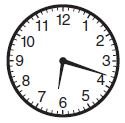_____ : _____ _____ (A.M./P.M.)

Explanation:
The times after midnight and before noon is written with A.M. At the early hours of the day sunrises which falls in the period of  12:00 at midnight to 12:00 noon, therefore the time 6:18 is considered to be A.M.

Question 4.
go for a walk_____ : _____ _____ (A.M./P.M.)

Explanation:
The times afternoon and before midnight is written with P.M. Since the people go for a walk to refresh their minds in the evening hours which falls in the period of 12:00 noon to 12:00 at midnight the time 3:55 is considered to be P.M.

Question 5.
go to school_____ : _____ _____ (A.M./P.M.)

Explanation:
The times after midnight and before noon is written with A.M. Since students go to the school in the morning hours which falls in the period of  12:00 at midnight to 12:00 noon, therefore, the time 8:10 is considered to be A.M.

Question 6.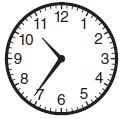_____ : _____ _____ (A.M./P.M.)

Explanation:
The times after midnight and before noon is written with A.M. Since students go to the art class in the morning hours which falls in the period of  12:00 at midnight to 12:00 noon, therefore, the time 10:36 is considered to be A.M.

Write the time. Use A.M. or P.M.

Question 7.
13 minutes after 5:00 in the morning
_____ : _____ _____ (A.M./P.M.)

Explanation:
The times after midnight and before noon is written with A.M. Since 5:00 in the morning falls in the period of 12:00 at midnight to 12:00 noon, therefore, the time is considered to be A.M.

Question 8.
19 minutes before 9:00 at night
_____ : _____ _____ (A.M./P.M.)

Explanation:
The times afternoon and before midnight is written with P.M.  Since 8:41 in the night time falls in the period of 12:00 noon to 12:00 at midnight, therefore, the time 8:41 is considered to be P.M.

Question 9.
quarter before midnight
_____ : _____ _____ (A.M./P.M.)

Explanation:
The times afternoon and before midnight is written with P.M. Since 11:45 in the night time falls in the period of 12:00 noon to 12:00 at midnight, therefore, the time 11:45 is considered to be P.M.

Question 10.
one-half hour after 4:00 in the morning
_____ : _____ _____ (A.M./P.M.)

Explanation:
One-half hour = 30 minutes So, by adding 30 minutes to 4:00 A.M. in the morning. We get the time to be 4:30 A.M.
The times after midnight and before noon is written with A.M.  Since 4:30 in the morning falls in the period of 12:00 at midnight to 12:00 noon, therefore, the time is considered to be A.M

Problem Solving

Question 11.
Jaime is in math class. What time is it? Use A.M. or P.M.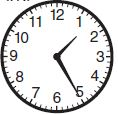_____ : _____ _____ (A.M./P.M.)

Explanation:
The times afternoon and before midnight is written with P.M. Since 1:25 in the afternoon hours fall in the period of 12:00 noon to 12:00 at midnight, therefore, the time1:25 is considered to be P.M.

Question 12.
Pete began practicing his trumpet at fifteen minutes past three. Write this time using A.M. or P.M.
_____ : _____ _____ (A.M./P.M.)

Explanation:
The times afternoon and before midnight is written with P.M. Since 3:15 in the evening hours fall in the period of 12:00 noon to 12:00 at midnight, therefore, the time 3:15 is considered to be P.M.

### A.M. and P.M. – Page No. 572

Lesson Check

Question 1.
Steven is doing his homework. What time is it? Use A.M. or P.M.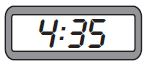Options:
a. 4:15 P.M.
b. 4:25 A.M.
c. 4:35 P.M.
d. 4:35 A.M.

Explanation:
The times afternoon and before midnight is written with P.M. Since 4:35 in the evening hours falls in the period of 12:00 noon to 12:00 at midnight, therefore, the time 4:35 is considered to be P.M.

After he finished breakfast, Mr. Edwards left for work fifteen minutes after seven. What time is this? Use A.M. or P.M.
Options:
a. 6:15 A.M.
b. 7:15 A.M.
c. 6:45 P.M.
d. 7:30 P.M.

Explanation:
The times after midnight and before noon is written with A.M. Since 7:15 in the morning falls in the period of 12:00 at midnight to 12:00 noon, therefore, the time 7:15 is considered to be A.M.

Spiral Review

Question 3.
Which division equation is related to the multiplication equation 4 × 6 = 24
Options:
a. 24 ÷ 8 = 3
b. 12 ÷ 3 = 4
c. 6 × 4 = 24
d. 24 ÷ 4 = 6

Answer: d. 24 ÷ 4 = 6

Explanation:
STEP 1 Draw 24 counters.
STEP 2 Make a group of 4 counters by drawing a circle around them. Continue circling groups of 4 until all 24 counters are in groups.
There are 6 groups of 24 counters.

Question 4.
There are 50 toothpicks in each box. Jaime buys 4 boxes for her party platter. How many toothpicks does Jaime buy in all?
Options:
a. 20
b. 54
c. 200
d. 2,000

Explanation:
Step1 let there be 50 toothpicks in each box.
Step2 the number of boxes required is 4
Step3 total number of toothpicks will be the number of boxes multiplied by the number of toothpicks in each box.
4 × 50=200

Question 5.
A pet store sold 145 bags of beef-flavored dog food and 263 bags of cheese-flavored dog food. How many bags of dog food were sold in all?
Options:
a. 118
b. 308
c. 408
d. 422

Explanation:
Total number of bags of dog food sold=145+263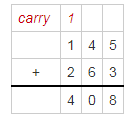Question 6.
Victoria and Melody are comparing fraction strips. Which statement is NOT correct?
Options:
a. $$\frac{1}{4}$$ < $$\frac{4}{4}$$
b. $$\frac{3}{6}$$ > $$\frac{4}{6}$$
c. $$\frac{2}{8}$$ > $$\frac{1}{8}$$
d. $$\frac{2}{3}$$ > $$\frac{3}{3}$$

Answer: b. $$\frac{3}{6}$$ > $$\frac{4}{6}$$

Explanation:

Take two blocks of the same size and both are made of 6 equal-size squares.
Compare 3/6 and 4/6.
The greater fraction will have the larger
Therefore, 3/6>4/6.

### Measure Time Intervals – Page No. 577

Find the elapsed time.

Question 1.
Start: 8:10 A.M. End: 8:45 A.M.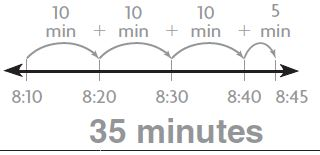Explanation:
STEP 1 Find 8:10 A.M. on the number line. Count on from 8:10 A.M. to 8:45 A.M. Draw marks and record the times on the number line. Then draw and label the jumps.
STEP 2 Add to find the total minutes from 8:10 A.M. to 8:45 A.M. From 8:10 A.M. to 8:45 A.M. is 35 minutes.
So, the elapsed time is 35 minutes

Question 2.
Start: 6:45 P.M. End: 6:54 P.M._______ minutes

Explanation:
STEP 1 Find the starting time on the clock.
STEP 2 Count the minutes by counting on by fives and ones to 6:54 P.M. Write the missing counting numbers next to the clock.
So, the elapsed time is 9 minutes.

Question 3.
Start: 3:00 P.M. End: 3:37 P.M._______ minutes

Explanation:
STEP 1 Find3:00 P.M. on the number line. Count on from 3:00 P.M. to 3:37 P.M. Draw marks and record the times on the number line. Then draw and label the jumps.
STEP 2 Add to find the total minutes from3:00 P.M. to 3:37 P.M. From 3:00 P.M. to 3:37 P.M. is 37 minutes.
So, the elapsed time is 37 minutes

Question 4.
Start: 10:05 A.M. End: 10:21 A.M.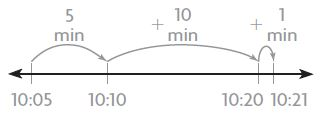_______ minutes

Explanation:
STEP 1 Find10:05 A.M. on the number line. Count on from 10:05 A.M. to 10:21 A.M. Draw marks and record the times on the number line. Then draw and label the jumps.
STEP 2 Add to find the total minutes from 10:05 A.M. to 10:21 A.M. From 10:05 A.M. to 10:21 A.M. is 16 minutes.
So, the elapsed time is 16 minutes

Question 5.
Start: 7:30 A.M. End: 7:53 A.M.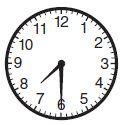_______ minutes

Explanation:
STEP 1 Find the starting time on the clock.
STEP 2 Count the minutes by counting on by fives and ones to7:53 A.M. Write the missing counting numbers next to the clock.
So, the elapsed time is 23 minutes.

Question 6.
Start: 5:20 A.M. End: 5:47 A.M._______ minutes

Explanation:
STEP 1 Find the starting time on the clock.
STEP 2 Count the minutes by counting on by fives and ones to 5:47 A.M. Write the missing counting numbers next to the clock.
So, the elapsed time is 27 minutes.

Problem Solving

Question 7.
A show at the museum starts at 7:40 P.M. and ends at 7:57 P.M. How long is the show?
_______ minutes

Explanation:
STEP 1 Find 7:40 P.M. on the number line. Count on from 7:40 P.M. to 7:57 P.M. Draw marks and record the times on the number line. Then draw and label the jumps.
STEP 2 Add to find the total minutes from 7:40 P.M. to 7:57 P.M. From 7:40 P.M. to 7:57 P.M. is 17 minutes.
So, the elapsed time is 17 minutes
The duration of the show is 17 minutes.

Question 8.
The first train leaves the station at 6:15 A.M. The second train leaves at 6:55 A.M. How much later does the second train leave the station?
_______ minutes

Explanation:
STEP 1 Find 6:15 A .M. on the number line. Count on from 6:15 A.M. to 6:55 A.M. Draw marks and record the times on the number line. Then draw and label the jumps.
STEP 2 Add to find the total minutes from 6:15 A.M. to 6:55 A.M. From 6:15 A .M. to 6:55 A.M. is 40 minutes.
So, the second train will leave the station in 40 minutes

### Measure Time Intervals – Page No. 578

Lesson Check

Question 1.
Marcus began playing basketball at 3:30 P.M. and stopped playing at 3:55 P.M. For how many minutes did he play basketball?
Options:
a. 25 minutes
b. 30 minutes
c. 55 minutes
d. 85 minutes

Explanation:
STEP 1 Find 3:30 P.M. on the number line. Count on from 3:30 P.M. to 3:55 P.M. Draw marks and record the times on the number line. Then draw and label the jumps.
STEP 2 Add to find the total minutes from3:30 P.M. to3:55 P.M. From 3:30 P.M. to 3:55 P.M. is 25 minutes.
So, the elapsed time is 25 minutes
Marcus played basketball for 25 minutes.

Question 2.
The school play started at 8:15 P.M. and ended at 8:56 P.M. How long was the school play?
Options:
a. 15 minutes
b. 31 minutes
c. 41 minutes
d. 56 minutes

Explanation:
STEP 1 Find 8:15 P.M. on the number line. Count on from 8:15 P.M. to 8:56 P.M. Draw marks and record the times on the number line. Then draw and label the jumps.
STEP 2 Add to find the total minutes from 8:15 P.M. to 8:56 P.M. From 8:15 P.M. to 8:56 P.M. is 41  minutes.
So, the elapsed time is 41 minutes
Therefore, the school play was for 41 minutes.

Spiral Review

Question 3.
Each car has 4 wheels. How many wheels will 7 cars have?
Options:
a. 11
b. 24
c. 27
d. 28

Explanation:
STEP 1 Draw 4 counters in each group.
STEP 2 Skip count to find how many wheels in all. Skip count by 4s until you say 7 numbers (groups)
There are 7 groups with 4 wheels in each group. So, there are 28 wheels in all.

Question 4.
Which number completes the equations?
3 × ■ = 27 27 ÷ 3 = ■
Options:
a. 6
b. 7
c. 8
d. 9

Explanation:
Count how many rows of 27 counters there are. There are  3 rows of 27 counters. The unknown factor is 9 (c0lumns). n = 9
3 × 9 = 27
STEP 1 Draw 27 counters.
STEP 2 Make a group of 3 counters by drawing a circle around them. Continue circling groups of 3 until all 27 counters are in groups.
There are 9 groups of 27 counters.

Question 5.
There are 20 napkins in each package. Kelli bought 8 packages for her party. How many napkins did Kelli buy in all?
Options:
a. 28
b. 40
c. 160
d. 180

Explanation:
Step1 let there be 20 napkins in each package.
Step2 the number of packages required is 8
Step3 total number of napkins will be the number of packages multiplied by the number of napkins in each package.
8 × 20=160

Question 6.
Mr. Martin drove 290 miles last week. This week he drove 125 miles more than last week. How many miles did Mr. Martin drive this week?
Options:
a. 125 miles
b. 165 miles
c. 315 miles
d. 415 miles

Explanation:
Total number of miles drove by Mr Martin in this week = 290+125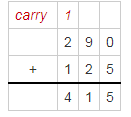### Use Time Intervals – Page No. 583

Find the starting time.

Question 1.
Ending time: 4:29 P.M.
Elapsed time: 55 minutes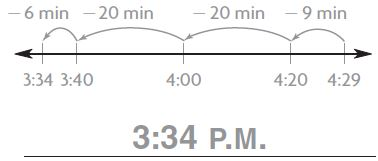Explanation:
STEP 1 Find the starting time on the number line
STEP 2 Count back on the number line to subtract the elapsed time. Draw and label the jumps to show the minutes.
STEP 3 Write the times below the number line.
You jumped back to 55 minutes
So, the starting time is 3:34 P.M.

Question 2.
Ending time: 10:08 A.M.
Elapsed time: 30 minutes______ : ______ ______ (A.M./P.M.)

Find the ending time.

Explanation:
STEP 1 Find the starting time on the clock.
STEP 2 Count back by fives for the elapsed time of 3o minutes. Write the missing counting numbers next to the clock.
So, the starting time is 9:38 A.M.

Question 3.
Starting time: 2:15 A.M.
Elapsed time: 45 minutes______ : ______ ______ (A.M./P.M.)

Explanation:
STEP 1 Find the ending time on the number line.
STEP 2 Count forward on the number line to add the elapsed time. Draw and label the jumps to show the minutes.
STEP 3 Write the times below the number line.
The jumps end at 3:00 A.M.
So, the ending time is 3:00 A.M.

Question 4.
Starting time: 6:57 P.M.
Elapsed time: 47 minutes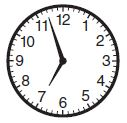______ : ______ ______ (A.M./P.M.)

Explanation:
STEP 1 Find the ending time on the clock.
STEP 2 Count on by fives and ones for the elapsed time of 47 minutes. Write the missing counting numbers next to the clock.
So, the ending time is 7:44 P.M.

Problem Solving

Question 5.
Jenny spent 35 minutes doing research on the Internet. She finished at 7:10 P.M. At what time did Jenny start her research?
______ : ______ ______ (A.M./P.M.)

Explanation:
STEP 1 Find the starting time on the clock.
STEP 2 Count back by fives for the elapsed time of 35 minutes. Write the missing counting numbers next to the clock.
So, the starting time is 6:35 A.M.

Question 6.
Clark left for school at 7:43 A.M. He got to school 36 minutes later. At what time did Clark get to school?
______ : ______ ______ (A.M./P.M.)

Explanation:
STEP 1 Find the ending time on the clock.
STEP 2 Count on by fives and ones for the elapsed time of 36 minutes. Write the missing counting numbers next to the clock.
So, the ending time is 8:19 A.M.

### Use Time Intervals – Page No. 584

Lesson Check

Question 1.
Cody and his friends started playing a game at 6:30 P.M. It took them 37 minutes to finish the game. At what time did they finish?
Options:
a. 5:07 P.M.
b. 5:53 P.M.
c. 6:53 P.M.
d. 7:07 P.M.

Explanation:
STEP 1 Find the ending time on the clock.
STEP 2 Count on by fives and ones for the elapsed time of 37 minutes. Write the missing counting numbers next to the clock.
So, the ending time is 7:07 P.M.

Question 2.
Delia worked for 45 minutes on her oil painting. She took a break at 10:35 A.M. At what time did Delia start working on the painting?
Options:
a. 9:40 A.M.
b. 9:50 A.M.
c. 11:20 A.M.
d. 11:30 A.M.

Explanation:
STEP 1 Find the starting time on the clock.
STEP 2 Count back by fives for the elapsed time of 45 minutes. Write the missing counting numbers next to the clock.
So, the starting time is 9:50 A.M.

Spiral Review

Question 3.
Sierra has 30 collector’s pins. She wants to put an equal number of pins in each of 5 boxes. How many pins should she put in each box?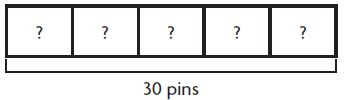Options:
a. 4
b. 5
c. 6
d. 8

Explanation:
Use 30 counters.
Take 5 boxes.
Place 1 counter at a time in each box until all 30 counters are used.
Place the rest of the counters till all the 30 counters (pins) are completed.
Total number of counters in each box is 6.

Question 4.
What time is shown on the clock?Options:
a. 1:24
b. 2:24
c. 4:12
d. 5:12

Explanation:
Count on by fives and ones from the 12 on the clock to where the minute hand is pointing. Write the missing counting numbers next to the clock.
Write: 2:24
• twenty-four minutes after two

Question 5.
Ricardo has 32 books to put on 4 shelves. He puts the same number of books on each shelf. How many books does Ricardo put on each shelf?
Options:
a. 6
b. 7
c. 8
d. 9

Explanation:
Use 32 counters. (books)
The number of shelves is 4.
Place 1 counter at a time in each shelf until all 32 counters are used.
Place the rest of the counters till all the 32 counters (books) are completed.
Total number of counters in each shelf is 8.

Question 6.
Jon started playing a computer game at 5:35 P.M. He finished the game at 5:52 P.M. How long did Jon play the game?
Options:
a. 17 minutes
b. 23 minutes
c. 25 minutes
d. 27 minutes

Explanation:
STEP 1 Find the ending time on the clock.
STEP 2 Count the minutes by counting on by fives and ones to 5:52 P.M. Write the missing counting numbers next to
the clock.
So, Jon has spent 17 minutes on the computer playing a game.

### Lesson 5 – Page No. 587

Share and Show

Question 1.
Patty went to the shopping mall at 11:30 a.m. She shopped for 25 minutes. She spent 40 minutes eating lunch.
Then she met a friend at a movie.
At what time did Patty meet her friend?
_______ : ________

Explanation:
The times afternoon and before midnight is written with P.M.
Count (25+40) minutes after 11:30 A.M.
Therefore the time at which Patty met her friend is 11:30 A.M.+1:05 hrs = 12:35 P.M.

Question 2.
What if Patty goes to the mall at 11:30 A.M. and meets a friend at a movie at 1:15 P.M.?
Patty wants to shop and have 45 minutes for lunch before meeting her friend.
How much time can Patty spend shopping?
_______ minutes

Explanation:
The times afternoon and before midnight are written with P.M.
Count 45 minutes before 1:15 P.M. (for lunch) which is equal to 12:30 P.M.
The difference between 12:30 P.M. and 11:30 A.M. gives the time spent by Patty in shopping.
STEP 1 Find 12:30 P.M. on the number line. Count on from 12:30 P.M. to 11:30 A.M. Draw marks and record the times on the number line. Then draw and label the jumps.
STEP 2 Add to find the total minutes from 12:30 P.M. to 11:30 A.M. From 12:30 P.M. to 11:30 A.M. is 60 minutes.
So, the time spent on shopping is 60 minutes

Go Math Grade 3 Chapter 10 Pdf Question 3.
Avery got on the bus at 1:10 p.m. The trip took 90 minutes. Then she walked for 32 minutes to get home. At what time did Avery arrive at home?
_______ : ________ P.M.

Explanation:
STEP 1 Find 1:10 P.M. on the number line. Count on from 1:10 P.M.
STEP2 Time spent in the trip= 90 minutes
Time Avery walked to get home = 32 minutes
STEP3 Then draw and label the jumps on the number line.
Add the total minutes; 90+32=122 minutes
So the time at which Avery arrives home is calculated by the cumulative jumps on the number line which is equal to 3:12 P.M.
Question 4.
Kyle and Josh have a total of 64 CDs. Kyle has 12 more CDs than Josh. How many CDs does each boy have?
Josh _______ CDs
Kyle _______ CDs

Kyle has 38 CDs

Explanation:
STEP 1 There are 64 counters. (CDs)
STEP2 Kyle has 12 CDs more than Josh. Place these 12 in a separate group. The leftover number of CDs is 64-12=52
STEP 3 Make 2 group and start placing the counters one after the other in each group until all the 52 counters are grouped
There are 2 groups of 26 counters.
Josh has 26 CDs while Kyle has 26+12 = 38 CDs

### Lesson 5 – Page No. 588

Question 5.
Jamal spent 60 minutes using the computer. He spent a half hour of the time playing games and the rest of the time researching his report. How many minutes did Jamal spend researching his report?
_______ minutes

Explanation: Jamal has spent half hour=30 minutes playing games and the rest of the time researching for his report.
STEP1 There are 60 counters(minutes).
STEP2 Remove the 30 counters which were used to play games
STEP3 The remaining number of counters in the box is 30
So the time spent on the report =30 minutes

Question 6.
When Caleb got home from school, he worked on his science project for 20 minutes. Then he studied for a test for 30 minutes. He finished at 4:35 p.m. At what time did Caleb get home from school?
_______ : _______ P.M.

Explanation:
STEP 1 Find the time on the number line when Caleb finished his homework.
STEP 2 Count back on the number line to subtract the elapsed time. Draw and label the jumps to show the minutes.
STEP 3 Write the times below the number line.
You jumped back to 30+20=50 minutes
Therefore Caleb got home from school at 3:45 P.M.

Question 7.
Miguel played video games each day for a week. On Monday, he scored 83 points. His score went up 5 points each day. On what day did Miguel score 103 points? Explain how you found your answer
_____________

Explanation:
STEP1 Miguel scored 83 points on Monday. His score went up 5 points each day.
STEP2 Add 5n counters to the points to reach 103
STEP3 Adding 5 points 5 times gives us the score to be 103 (83+5*5)
STEP4 Therefore Miguel takes 5 days to score 103 points

Question 8.
When Laura arrived at the library, she spent 40 minutes reading a book. Then she spent 15 minutes reading a magazine. She left the library at 4:15 p.m. Circle the time that makes the sentence true.
Laura arrived at the library at:
_______ : _______ P.M.

Explanation:
STEP 1 Find the time on the number line when Laura arrived at the library.
STEP 2 Count back on the number line to subtract the elapsed time. Draw and label the jumps to show the minutes.
STEP 3 Write the times below the number line.
You jumped back to 40+15=55 minutes
Therefore Laura arrived at the library at 3:20 P.M.

### Problem Solving Time Intervals – Page No. 589

Solve each problem. Show your work.

Question 1.
Hannah wants to meet her friends downtown. Before leaving home, she does chores for 60 minutes and eats lunch for 20 minutes. The walk downtown takes 15 minutes. Hannah starts her chores at 11:45 A.M. At what time does she meet her friends?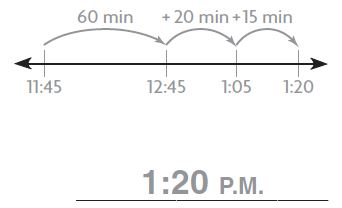Explanation:
STEP 1 Find the ending time on the clock, the time at which Hannah meets her friends.
STEP 2 Count back by fives for the elapsed time of 95 minutes. Write the missing counting numbers next to the clock.
So, the ending time is 1:20 P.M.

Question 2.
Katie practiced the flute for 45 minutes. Then she ate a snack for 15 minutes. Next, she watched television for 30 minutes, until 6:00 P.M. At what time did Katie start practicing the flute?
_______ : ________ (A.M./P.M.)

Explanation:
STEP 1 Find the starting time on the clock.
STEP 2 Count back by fives for the elapsed time of 90 minutes. Write the missing counting numbers next to the clock.
So, the starting time is 4:30 P.M.

Question 3.
Nick gets out of school at 2:25 P.M. He has a 15-minute ride home on the bus. Next, he goes on a 30-minute bike ride. Then he spends 55 minutes doing homework. At what time does Nick finish his homework?
_______ : ________ (A.M./P.M.)

Explanation:
STEP 1 Find the ending time on the clock, the time at which Nick finishes his work.
STEP 2 Count back by fives for the elapsed time of 100 minutes. Write the missing counting numbers next to the clock.
So, the ending time is 4:05 P.M.

Question 4.
The third-grade class is going on a field trip by bus to the museum. The bus leaves the school at 9:45 a.m The bus ride takes 47 minutes. At what time does the bus arrive at the museum?
_______ : ________ (A.M./P.M.)

Explanation:
STEP 1 Find the ending time on the clock, the time at which the students arrive at the museum.
STEP 2 Count back by fives for the elapsed time of 47 minutes. Write the missing counting numbers next to the clock.
So, the ending time is 10:32 A.M.

### Problem Solving Time Intervals – Page No. 590

Lesson Check

Question 1.
Gloria went to the mall and spent 50 minutes shopping. Then she had lunch for 30 minutes. If Gloria arrived at the mall at 11:00 A.M., at what time did she finish lunch?
Options:
a. 11:30 A.M.
b. 11:50 A.M.
c. 12:20 P.M.
d. 12:30 P.M.

Explanation:
STEP 1 Find the ending time on the clock, the time at which Gloria finish her lunch.
STEP 2 Count back by fives for the elapsed time of 80 minutes. Write the missing counting numbers next to the clock.
So, the ending time is 12:20 P.M.

Question 2.
The ball game begins at 2:00 P.M. It takes Ying 30 minutes to get to the ballpark. At what time should Ying leave home to get to the game 30 minutes before it starts?
Options:
a. 12:30 P.M.
b. 1:00 P.M.
c. 1:30 P.M.
d. 3:00 P.M.

Explanation:
STEP 1 Find the starting time on the clock.
STEP 2 Count back by fives for the elapsed time of 60 minutes. Write the missing counting numbers next to the clock.
So, the starting time is 1:00 P.M.

Spiral Review

Question 3.
Which lists the fractions in order from least to greatest?
Options:
a. $$\frac{2}{8}$$, $$\frac{2}{4}$$, $$\frac{2}{6}$$
b. $$\frac{2}{4}$$, $$\frac{2}{8}$$, $$\frac{2}{6}$$
c. $$\frac{2}{8}$$, $$\frac{2}{6}$$, $$\frac{2}{4}$$
d. $$\frac{2}{4}$$, $$\frac{2}{6}$$, $$\frac{2}{8}$$

Answer: c. $$\frac{2}{8}$$, $$\frac{2}{6}$$, $$\frac{2}{4}$$

Explanation:
When the numerators are the same, think about the  pieces to compare and order fractions. So, the order from least to greatest  is $$\frac{2}{8}$$, $$\frac{2}{6}$$, $$\frac{2}{4}$$

Question 4.
Find the unknown factor.
6 × ■ = 36
Options:
a. 4
b. 6
c. 7
d. 8

Explanation:
Count how many rows of 36 counters there are.
There are  6 rows of 36 counters. The unknown factor is 6 (c0lumns). n = 6
6× 6 = 36

Question 5.
There were 405 books on the library shelf. Some books were checked out. Now there are 215 books left on the shelf. How many books were checked out?
Options:
a. 620
b. 220
c. 210
d. 190

Explanation:
STEP1 Let there be 405 books in a shelf of Library.
STEP2 Some books were checked out.
STEP3 Number of books left in the shelf are 215.
STEP4 Take a box filled with 405 counters.
STEP5 Place 215 counters in another box from the initial 405 counters.
STEP6 The number of counters left in the first box is equal to the books checked out.
405-215=190

Question 6.
Savannah has 48 photos. She places 8 photos on each page of her photo album. How many pages in the album does she use?
Options:
a. 5
b. 6
c. 7
d. 9

Explanation:
STEP 1 Draw 48 counters.
STEP 2 Make a group of 8 counters by drawing a circle around them. Continue circling groups of 8 until all 48 counters are in groups.
There are 6 groups of 48 counters.

### Mid -Chapter Checkpoint – Page No. 591

Vocabulary

Choose the best term from the box.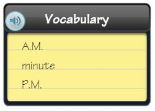Question 1.
In one __________, the minute hand moves from one mark to the next on a clock.

Explanation: The minute hand moves after every 60 seconds = 1 Minute to the next mark on the clock.

Question 2.
The times afternoon and before midnight are written with __________ .
__________

Explanation:
The times afternoon and before midnight are written with P.M.

Concepts and Skills

Write the time for the activity. Use A.M. or P.M.

Question 3.
play ball______ : ______ ______ (A.M./P.M.)

Explanation:
The times afternoon and before midnight is written with P.M. Since we play ball in the evening, the time is considered to be P.M.

Question 4.
eat breakfast______ : ______ ______ (A.M./P.M.)

Explanation:
The times after midnight and before noon is written with A.M. Since we have our breakfast in the morning, the time is considered to be  A.M.

Question 5.
do homework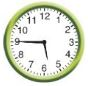______ : ______ ______ (A.M./P.M.)

Explanation:
The times afternoon and before midnight is written with P.M. Since we do our homework in the evening, the time is considered to be P.M.

Question 6.
sleep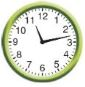______ : ______ ______ (A.M./P.M.)

Explanation:
The times afternoon and before midnight is written with P.M. Since we go to sleep in the night hours, the time is considered to be P.M.

Find the elapsed time.

Question 7.
Start: 10:05 A.M. End: 10:50 A.M._______ minutes

Explanation:
STEP 1 Find 10:05 A.M. on the number line. Count from 10:05 A.M. to 10:50 A.M. Draw marks and record the times on the number line. Then draw and label the jumps.
STEP 2 Add to find the total minutes from 10:05 A.M. to 10:50 A.M. From 10:05 A.M. to 10:50 A.M. is 45 minutes.
So, the elapsed time is 45 minutes

Question 8.
Start: 5:30 P.M.
End: 5:49 P.M._______ minutes

Explanation:
STEP 1 Find the starting time on the clock that is 5:30 P.M.
STEP 2 Count the minutes by counting on by fives and ones to 5:49 P.M. Write the missing counting numbers next to the clock.
So, the elapsed time is 19 minutes.

Find the starting time or the ending time.

Question 9.
Elapsed time: 50 minutes
Ending time: 9:05 A.M.Starting time: ______ : ______ A.M.

Explanation:
STEP 1 Find the starting time on the number line
STEP 2 Count back on the number line to subtract the elapsed time. Draw and label the jumps to show the minutes.
STEP 3 Write the times below the number line.
You jumped back to 50 minutes
So, the starting time is 8:15 A.M.

Question 10.
Starting time: 2:46 P.M.
Elapsed time: 15 minutesEnding time: ______ : ______ P.M.

Explanation:
STEP 1 Find the ending time on the clock
STEP 2 Count back by fives for the elapsed time of 15 minutes. Write the missing counting numbers next to the clock.
So, the ending time is 3:01 P.M.

### Mid -Chapter Checkpoint – Page No. 592

Question 11.
Veronica started walking to school at 7:45 A.M. She 0 arrived at school 23 minutes later. At what time did Veronica arrive at school?
______ : ______ ______

Explanation:
STEP 1 Find the ending time on the number line.
STEP 2 Count forward on the number line to add the elapsed time. Draw and label the jumps to show the minutes.
STEP 3 Write the times below the number line.
The jumps end at 8:08 A.M.
So, the ending time is 8:08 A.M.

Question 12.
The clock shows the time the art class ends. At what time does it end? If the class started 37 minutes before the time shown, at what time did the class start?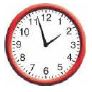Type below:
____________

Answer: Time at which the class ends= 1:57 P.M.
Time at which the class starts= 1:20 P.M.

Explanation:
Count by fives and ones from the 12 on the clock back to where the minute hand is pointing. Write the missing counting numbers next to the clock.
Write: 1:57 P.M.
Three minutes before two
STEP 1 Find the starting time on the clock.
STEP 2 Count back by fives for the elapsed time of 37 minutes. Write the missing counting numbers next to the clock.
So, the starting time is 1:20 P.M.

Question 13.
Matt went to his friend’s house. He arrived at 5:10 P.M. He left at 5:37 P.M. How long was Matt at his friend’s house?
______ minutes

Explanation:
STEP 1 Find 5:10 P.M. on the number line. Count on from 5:10 P.M. to 5:37 P.M. Draw marks and record the times on the number line. Then draw and label the jumps.
STEP 2 Add to find the total minutes from 5:10 P.M. to 5:37 P.M. From 5:10 P.M. to 5:37 P.M. is 27 minutes.
So, the elapsed time is 27 minutes

Go Math Grade 3 Measure Length Lesson 10.6 Question 14.
Brenda’s train leaves at 7:30 A.M. She needs to arrive 10 minutes early to buy her ticket. It takes her 20 minutes to get to the train station. At what time should Brenda leave her house?
______ : ______ A.M.

Explanation:
STEP 1 Find the starting time on the number line
STEP 2 Count back on the number line to subtract the elapsed time. Draw and label the jumps to show the minutes.
STEP 3 Write the times below the number line.
You jumped back to 30 minutes
So, the starting time is 7:00 A.M.

Question 15.
Write the time you get home from school.
Type below:
____________

Explanation:
The times afternoon and before midnight is written with P.M. Since we get home from school in the evening, the time is considered to be P.M.

### Measure Length – Page No. 597

Measure the length to the nearest half inch.

Question 1.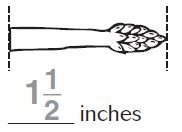Explanation:
Line up the left end of the glue stick with the zero mark on the ruler.
The right end of the glue stick is between the half-inch marks for 1 and 2.
The mark that is closest to the right end of the glue stick is for one and a half inches.
So, the length of the glue stick to the nearest half-inch is 1 1/2 inches.

Question 2.______ inches

Explanation:
Line up the left end of the glue stick with the zero mark on the ruler.
The right end of the glue stick is between the half-inch marks for 1 and 3.
The mark that is closest to the right end of the glue stick is for three inches.
So, the length of the glue stick to the nearest half-inch is 3 inches.

Question 3.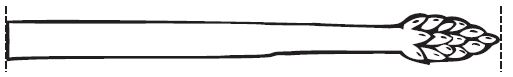______ $$\frac{□}{□}$$ inches

Explanation:
Line up the left end of the glue stick with the zero mark on the ruler.
The right end of the glue stick is between the half-inch marks for4 and 5.
The mark that is closest to the right end of the glue stick is for four and a half  inches.
So, the length of the glue stick to the nearest half-inch is 4 1/2 inches.

Measure the length to the nearest fourth inch.

Question 4.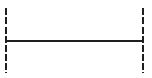______ $$\frac{□}{□}$$ inches

Explanation:
Line up the left end of the paper clip with the zero mark on the ruler.
The right end of the paper clip is between the fourth-inch marks for 1 and2.
The mark that is closest to the right end of the paper clip is for 1 1/4 inches.
So, the length of the paper clip to the nearest fourth inch is 1 1/4 inches.

Question 5.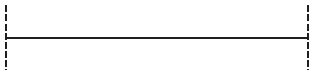______ $$\frac{□}{□}$$ inches

Explanation:
Line up the left end of the paper clip with the zero mark on the ruler.
The right end of the paper clip is between the fourth-inch marks for 2 and 3.
The mark that is closest to the right end of the paper clip is for 2 3/4 inches.
So, the length of the paper clip to the nearest fourth inch is 2 3/4 inches.

Question 6.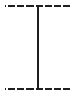$$\frac{□}{□}$$ inch

Explanation:
Line up the left end of the paper clip with the zero mark on the ruler.
The right end of the paper clip is between the fourth-inch marks for 0 and1.
The mark that is closest to the right end of the paper clip is for 3/4 inches.
So, the length of the paper clip to the nearest fourth inch is 3/4 inches.

Question 7.______ inches

Explanation:
Line up the left end of the paper clip with the zero mark on the ruler.
The right end of the paper clip is between the fourth-inch marks for 1 and2.
The mark that is closest to the right end of the paper clip is for 2 inches.
So, the length of the paper clip to the nearest fourth inch is 2 inches.

Problem Solving

Use a separate sheet of paper for 8.

Question 8.
Draw 8 lines that are between 1 inch and 3 inches long. Measure each line to the nearest fourth inch, and make a line plot.

Explanation:

Question 9.
The tail on Alex’s dog is 5$$\frac{1}{4}$$ inches long. This length is between which two inch-marks on a ruler?
Between ______ and ______ inch-marks

Answer: Between 5 and 6 inches

Explanation:
Line up the left end of the paper clip with the zero mark on the ruler.
The right end of the paper clip is between the fourth-inch marks for 5 and 6.
The mark that is closest to the right end of the paper clip is for 5 1/4 inches.
So, the length of the paper clip to the nearest fourth inch is 5 1/4 inches.

### Measure Length – Page No. 598

Lesson Check

Question 1.
What is the length of the eraser to the nearest half inch?Options:
a. $$\frac{1}{2}$$ inch
b. 1 inch
c. 1 $$\frac{1}{2}$$ inch
d. 2 inch

Answer: c. 1 $$\frac{1}{2}$$ inch

Explanation:
Line up the left end of the glue stick with the zero mark on the ruler.
The right end of the glue stick is between the half-inch marks for 1 and 2.
The mark that is closest to the right end of the glue stick is for one and a half inches.
So, the length of the glue stick to the nearest half-inch is 1 1/2 inches.

Question 2.
What is the length of the leaf to the nearest fourth inch?Options:
a. 1 $$\frac{1}{2}$$ inches
b. 1 $$\frac{3}{4}$$ inches
c. 2 inches
d. 2 $$\frac{1}{4}$$ inches

Explanation:
Line up the left end of the paper clip with the zero mark on the ruler.
The right end of the paper clip is between the fourth-inch marks for 1 and 2.
The mark that is closest to the right end of the paper clip is for 2 inches.
So, the length of the paper clip to the nearest fourth inch is 2 inches.

Spiral Review

Question 3.
Which equation is NOT included in the same set of related facts as 6 × 8 = 48?
Options:
a. 8 × 6 = 48
b. 8 × 8 = 64
c. 48 ÷ 6 = 8
d. 48 × 8 = 6

Answer: b. 8 × 8 = 64

Explanation:
STEP 1 Use 8 tiles to make an array with 6 equal rows.
Draw the rest of the tiles.
There are 8 tiles in each row.
Write a division equation for the array using the total number of tiles as the dividend and the number of rows as the divisor.
48÷ 6= 8
So, 48 ÷ 8 = 6, 8 × 6 = 48,
and 6 ×8  = 48 are related facts.

Question 4.
Brooke says there are 49 days until July 4. There are 7 days in a week. In how many weeks will it be July 4?
Options:
a. 9 weeks
b. 8 weeks
c. 7 weeks
d. 6 weeks

Explanation:
Draw 1 tile in each of 7 rows.
Continue drawing 1 tile in each of the 7 rows until all 49 tiles are drawn.
Count the number of tiles in each row.
There are 7 tiles in each row.
So, there are 7 weeks till July 4.

Question 5.
It is 20 minutes before 8:00 in the morning. Which is the correct way to write that time?
Options:
a. 7:40 A.M.
b. 7:40 P.M.
c. 8:20 A.M.
d. 8:40 A.M.

Explanation:
Count by fives and ones from the 12 on the clock back to where the minute hand is pointing. Write the missing counting numbers next to the clock.
Write: 7:40 A.M.
Twenty minutes before eight

Question 6.
Marcy played the piano for 45 minutes. She stopped playing at 4:15 P.M. At what time did she start playing the piano?
Options:
a. 3:00 P.M.
b. 3:30 P.M.
c. 4:45 P.M.
d. 5:00 P.M.

Explanation:
STEP 1 Find the starting time on the number line
STEP 2 Count back on the number line to subtract the elapsed time. Draw and label the jumps to show the minutes.
STEP 3 Write the times below the number line.
You jumped back to 45 minutes
So, the starting time is 3:30 P.M.

### Estimate and Measure Liquid Volume – Page No. 603

Estimate how much liquid volume there will be when the container is filled. Write more than 1 liter, about 1 liter, or less than 1 liter.

Question 1.
large milk container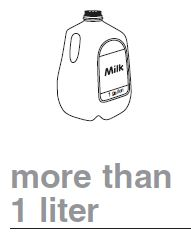Explanation:
Pour 1 liter of milk into one of the large containers. Repeat until the container is full. Record the number of liters you poured. This proves that the container can hold more than one liter.

Question 2.
small milk container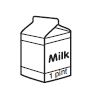____________

Explanation:
Pour 1 liter of milk into one of the small containers. There is some quantity of milk left in the pack of one liter, this proves that the container can hold less than one liter.

Question 3.
water bottle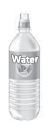____________

Explanation:
A water bottle holds about 1 liter.

Question 4.
spoonful of water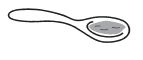____________

Explanation:
Pour 100 ml of water into a spoon. The excess water flow out, this proves that the spoon can hold less than one liter.

Question 5.
bathtub filled halfway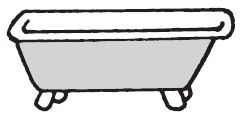____________

Explanation:
Pour 1 liter of water into the bathtub. Repeat until the tub is full. Record the number of liters you poured.This proves that the container can hold more than one liter.

Question 6.
filled eyedropper____________

Explanation:
Place the eyedropper in a bottle of the quantity, one liter. The eyedropper picks only few drops of the water, this proves that the eyedropper can hold less than one liter.

Problem Solving

Use the pictures for 7–8. Alan pours water into four glasses that are the same size.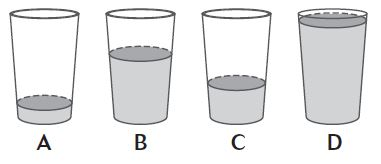Question 7.
Which glass has the most amount of water?
_______

Explanation:
It can be observed that glass D is almost completely filled. So, glass D most amount of water.

Question 8.
Which glass has the least amount of water?
_______

Explanation:
It can be observed that glass A contains the least amount of water. So, glass A has the least amount of water.

### Estimate and Measure Liquid Volume – Page No. 604

Lesson Check

Question 1.
Felicia filled the bathroom sink with water. About how much water does she put in the sink?
Options:
b. more than 1 liter
c. a little less than 1 liter
d. much less than 1 liter

Answer: b. more than 1 liter

Explanation:
Pour one liter of water into the sink. By repeating the same process we can say that, a sink can hold more than one liter of water. Hence it contains more than one liter of water.

Question 2.
Kyle needed about 1 liter of water to fill a container. Which container did Kyle most likely fill?
Options:
a. a small glass
b. a spoon
c. a large pail
d. a vase

Explanation:
A vase holds about 1 liter while a small glass, a spoon can only hold small quantity of water. A large pail holds water more than one liter.

Spiral Review

Question 3.
Cecil had 6 ice cubes. He put 1 ice cube in each glass. In how many glasses did Cecil put ice cubes?
Options:
a. 6
b. 5
c. 1
d. 0

Explanation:
If there is the same number of ice cubes and glasses, then 1 ice cube goes in each glass. Since each ice cube is to be placed in each glass.
6÷ 1 = 6
Number of glasses=6

Question 4.
Juan has 12 muffins. He puts $$\frac{1}{4}$$ of the muffins in a bag. How many muffins does Juan put in the bag?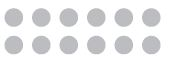Options:
a. 2
b. 3
c. 4
d. 5

Explanation:
Put 12 counters(muffins) on the table.
Since you want to find 1/4 of the group, there should be equal groups of muffins.
Circle one of the groups to show 1/4th part of the muffins. Then count the number of counters in that group.
There are 3 counters in 1 group. 1/4 of 12 = 3
So,  Juan has put 3 muffins in his bag.

Estimate and Measure Mass Lesson 10.8 Question 5.
Which is one way to read the time shown on the clock?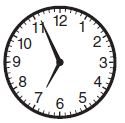Options:
a. 4 minutes before 7
b. 26 minutes before 11
c. 54 minutes after 6
d. 56 minutes after 7

Answer: a. 4 minutes before 7

Explanation:
Count by fives and ones from the 12 on the clock back to where the minute hand is pointing. Write the missing counting numbers next to the clock.
Write: 6:56
four minutes before seven

Question 6.
Julianne drew the line segment below. Use your ruler to measure the segment to the nearest fourth inch.Options:
a. $$\frac{3}{4}$$ inch
b. 1 $$\frac{1}{4}$$ inches
c. 1 $$\frac{1}{2}$$ inches
d. 1 $$\frac{3}{4}$$ inches

Answer: d. 1 $$\frac{3}{4}$$ inches

Explanation:
Line up the left end of the paper clip with the zero mark on the ruler.
The right end of the paper clip is between the fourth-inch marks for 1 and 2.
The mark that is closest to the right end of the paper clip is for 1 $$\frac{3}{4}$$ inches.
So, the length of the paper clip to the nearest fourth inch is 1 $$\frac{3}{4}$$ inches.

### Estimate and Measure Liquid Volume – Page No. 609

Choose the unit you would use to measure the mass. Write a gram or kilogram.

Question 1.
CDgram

Explanation:
The gram (g) is the basic metric unit for measuring mass or the amount of matter in an object. Mass can also be measured by using the metric unit kilogram (kg).
A small CD has a mass measured in grams.

Question 2.
boy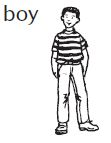________

Explanation:
A boy’s mass must be measured in kilograms, the metric unit. Since gram is the basic unit of mass and this measuring mass is used to measure small items such as CDs, paper pins, ice cream sticks etc.

Question 3.
bag of sugar________

Explanation:
A bag contains more than or equal to 1000 grams of measuring mass.
1000grams=1kilogram
Hence we can say that the sugar is measured in kilograms.

Question 4.
lion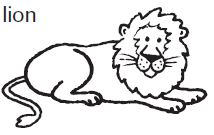________

Explanation: Lion is a huge animal with muscles and hard bones. Hence, Lion is measured in kilograms.

Question 5.
paper clip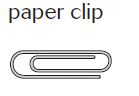________

Explanation:
A paper clip is light measured clip its mass is about 1 gram.

Question 6.
empty plastic bottle________

Explanation:
The amount of water in the bottle justifies the mass/volume of the bottle. Since the bottle is empty it is only measured in grams.

Compare the masses of the objects. Write is less than,
is the same as, or is more than.

Question 7.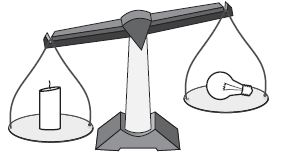The mass of the candle ________ the mass of the light bulb.

Explanation:
Both candle and bulb measure in grams comparatively candle seems to have more mass than bulb.
Therefore we can say that mass of candle is more than a mass of bulb.

Question 8.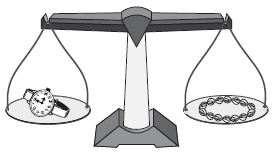The mass of the watch ________ the mass of the necklace.

Explanation:
Both the watch and the necklace seem to have same amount of mass in grams.

Problem Solving

Question 9.
A red ball has a mass that is less than 1 kilogram. A blue ball has a mass of 1 kilogram. Is the mass of the blue ball more than or less than the mass of the red ball?
The mass of the blue ball is ________ the mass of the red ball

Explanation:
Blue ball has a mass of 1 kilogram which more than that of the red ball because it has a mass less than 1 kilogram.

Question 10.
Brock’s dog is a collie. To find the mass of his dog, should Brock use grams or kilograms?
__________

Explanation:

### Estimate and Measure Liquid Volume – Page No. 610

Lesson Check

Question 1.
Which unit of measure would you use to measure the mass of a grape?
Options:
a. gram
b. inch
c. kilogram
d. meter

Explanation:
A bunch of grapes are measured in kilograms while a single grape is measured in grams.

Question 2.
Elsie wants to find the mass of her pony. Which unit should she use?
Options:
a. gram
b. liter
c. kilogram
d. centimeter

Explanation:
The mass of the pony is calculated in kilogram because the cluster of grams is called as kilogram.

Spiral Review

Question 3.
Marsie blew up 24 balloons. She tied the balloons together in groups of 4. How many groups did Marsie make?
Options:
a. 5
b. 6
c. 7
d. 8

Explanation:
STEP 1 Let 24 counters be ballons.
STEP 2 Make a group of 4 counters tied together into a group. Continue making groups of 4 until all 24 counters are in groups.
There are 6 groups of 24 counters.

Question 4.
Clark used the order of operations to find the unknown number in 15 − 12 ÷ 3 = n. What is the value of the unknown number?
Options:
a. 1
b. 6
c. 9
d. 11

Explanation:
STEP 1 Let there be 12 counters in a box.
STEP 2 Make a group of 3 counters by drawing a circle around them. Continue circling groups of 3 until all 12 counters are in groups.
There are 4 groups of 12 counters.
STEP3 There are 15 counters in a box
STEP4 Remove 4 counters from the box, then only 11 counters are left in the box.

Use the pictures for 5–6. Ralph pours juice into four bottles that are the same size.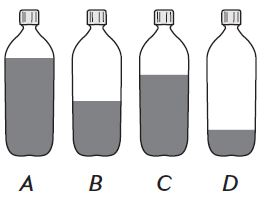Question 5.
Which bottle has the most amount of juice?
Options:
a. Bottle A
b. Bottle B
c. Bottle C
d. Bottle D

Explanation:
Comparatively in all the four bottles bottle A is almost completely filled. Therefore bottle A has the most amount of juice.

Question 6.
Which bottle has the least amount of juice?
Options:
a. Bottle A
b. Bottle B
c. Bottle C
d. Bottle D

Explanation:
Comparatively in all the four bottles bottle D has the least amount of juice. Therefore bottle A has the least amount of juice.

### Solve Problems About Liquid Volume and Mass – Page No. 615

Write an equation and solve the problem.

Question 1.
Luis was served 145 grams of meat and 217 grams of vegetables at a meal. What was the total mass of the meat and the vegetables?
Think: Add to find how much in all.
145 + 217 = _______ grams

Answer: 145 + 217 = 362 grams

Explanation:
STEP 1 Place 145 grams mass of meat.
STEP 2 Place 217 grams mass of vegetables at a meal .
STEP 3 To find the total mass of the meat and the vegetables is 362 grams.
So, the total mass of the meat and the vegetables is 362 grams.

Question 2.
The gas tank of a riding mower holds 5 liters of gas. How many 5-liter gas tanks can you fill from a full 20-liter gas can?
_______ ÷ _______ = _______ 5-liter gas tanks

Answer: 20 ÷ 5 = 4 gas tanks

Explanation:
STEP1 A single gas tank of a riding mower holds 5 liters of gas.
STEP2 Quantity of gas given to fill in the tanks is 20 liters.
STEP3 To calculate the number of tanks, we should make groups of 5 liters of gas such that the total quantity of 20 liters is completed
STEP4 The number of gas tanks is the number of counters in which the 20 liter of gas is filled.
Therefore the number of tanks are 4

Question 3.
To make a lemon-lime drink, Mac mixed 4 liters of lemonade with 2 liters of limeade. How much lemon-lime drink did Mac make?
_______ + _______ = _______ liters lemon-lime

Answer: 4 + 2 = 6 liters

Explanation:
STEP 1 Place 4 liters of lemonade for preparing the lemon-lime drink.
STEP 2 Place 2 liters of limeade for preparing the lemon-lime drink.
STEP 3Mass of lemon-lime drink made by Mac is 4+2=6 liters.
So, the total mass of the lemon-lime drink made is 6 liters.

Question 4.
A nickel has a mass of 5 grams. There are 40 nickels in a roll of nickels. What is the mass of a roll of nickels?
_______ × _______ = _______grams

Answer: 5 × 40 = 200 grams

Explanation:
STEP1 Given that there are 40 nickels in a roll of nickels.
STEP2 Each nickel has a mass of 5 grams.
STEP3 Place the counters(nickels) in rows.
STEP4 Find the mass of a roll of nickels.
STEP5 Mass of a roll of nickels=5×40
STEP6 Place 5 boxes each containing 40 counters. Add the counters in the boxes, total number of counters is equal to 200.

Question 5.
Four families share a basket of 16 kilograms of apples equally. How many kilograms of apples does each family get?
_______ ÷ _______ = _______ kilograms

Answer: 20 ÷ 5 = 4

Explanation:
STEP1 Total number of apples =16
STEP2 Number of families =4
STEP3 Number of apples each family shared=16÷4
=4 apples

Question 6.
For a party, Julia made 12 liters of fruit punch. There were 3 liters of fruit punch left after the party. How much fruit punch did the people drink at the party?
_______ – _______ = _______ liters

Answer: 12 – 3 = 9 liters

Explanation:
STEP1 Quantity of fruit punch made by Julia=12 liters
STEP2 Quantity of fruit punch left after the party=3 liters
STEP3 Quantity of fruit punch the people drank in the party=12-3 = 9 liters

Problem Solving

Question 7.
Zoe’s fish tank holds 27 liters of water. She uses a 3-liter container to fill the tank. How many times does she have to fill the 3-liter container in order to fill her fish tank?
_______ times

Explanation:
STEP1 Quantity of water Zoe’s fish tank holds=27 liters
STEP2  Zoe has a 3-liter container to fill the tank.
STEP3 Total number of times  she has to fill the 3-liter container in order to fill her fish tank=27÷3=9 times

Question 8.
Adrian’s backpack has a mass of 15 kilograms. Theresa’s backpack has a mass of 8 kilograms. What is the total mass of both backpacks?
15 + 8 = _______ kilograms

Explanation:
STEP1 Mass of Adrian’s backpack = 15 kilograms
STEP2 Mass of Theresa’s backpack= 8 kilograms
STEP3  Total mass of both backpacks=15+8=23 kilograms

### Solve Problems About Liquid Volume and Mass – Page No. 616

Lesson Check

Question 1.
Mickey’s beagle has a mass of 15 kilograms. His dachshund has a mass of 13 kilograms. What is the combined mass of the two dogs?
Options:
a. 2 kilograms
b. 18 kilograms
c. 23 kilograms
d. 28 kilograms

Explanation:
Mickey has 2 dogs beagle and dachshund
STEP1 Mass of Beagle=15 kilograms
STEP2 Mass of dachshund= 13 kilograms
STEP3 Combined mass of the two dogs= 15+13=28 kilograms

Question 2.
Lois put 8 liters of water in a bucket for her pony. At the end of the day, there were 2 liters of water left. How much water did the pony drink?
Options:
a. 4 liters
b. 6 liters
c. 10 liters
d. 16 liters

Explanation:
STEP1 Quantity of the water put in the bucket by Lois=8 liters
STEP2 Quantity of the water left in the bucket=2 liters
STEP3 Quantity of the water drank by pony= 8-2 = 6 liters

Spiral Review

Question 3.
Josiah has 3 packs of toy animals. Each pack has the same number of animals. Josiah gives 6 animals to his sister Stephanie. Then Josiah has 9 animals left. How many animals were in each pack?
Options:
a. 1
b. 3
c. 5
d. 6

Explanation:
STEP1 Number of packs Josiah has =3
STEP2 Number of animals given by Josiah to his sister =6
STEP3 Total number of animals = 6+9=15
According to the problem,
Each pack has the same number of animals.
3× ■ = 15
Count how many rows of 15 counters there are. There are  3 rows of 15 counters. The unknown factor is 5 (c0lumns). n = 5
3 × 5 = 15

Question 4.
Tom jogged $$\frac{3}{10}$$ mile, Betsy jogged $$\frac{5}{10}$$ mile, and Sue jogged $$\frac{2}{10}$$ mile. Who jogged a longer distance than $$\frac{4}{10}$$ mile?
Options:
a. Betsy
b. Sue
c. Tom
d. None

Explanation:
When the numerators are the same, think about the of the pieces to compare and order fractions. So, the order from greatest to least gives, the greatest fraction and the person who jogged a longer distance than $$\frac{4}{10}$$ mile.
$$\frac{5}{10}$$>$$\frac{3}{10}$$>$$\frac{2}{10}$$
Therefore Betsy jogged a longer distance than $$\frac{4}{10}$$ mile.

Question 5.
Bob started mowing at 9:55 A.M. It took him 25 minutes to mow the front yard and 45 minutes to mow the back yard. At what time did Bob finish mowing?
Options:
a. 10:20 A.M.
b. 10:55 A.M.
c. 11:05 A.M.
d. 11:20 A.M.

Explanation:
STEP1 Time Bob spent in mowing=25+45=70 minutes
STEP2 Finding the time at which Bob finished mowing
STEP3 Find the ending time on the number line.
STEP4 Count forward on the number line to add the elapsed time. Draw and label the jumps to show the minutes.
STEP5 Write the times below the number line.
The jumps end at 11:05 A.M.
So, the ending time is 11:05 A.M.

Question 6.
Juliana wants to find the mass of a watermelon. Which unit should she use?
Options:
a. gram
b. kilogram
c. liter
d. meter

Explanation:
Mass is measured in grams and kilograms small quantities like paper clips, pins are measured in grams while large quantities are measured in kilograms. Therefore watermelon is measured in kilogram.

### Review/Test – Page No. 617

Question 1.
Yul and Sarah’s art class started at 11:25 a.m. The class lasted 30 minutes. Yul left when the class was done. Sarah stayed an extra 5 minutes to talk with the teacher and then left.
Write the time that each student left. Explain how you found each time.
Yul: _______ A.M.
Sarah: ______ P.M.

Sarah: 12:05 P.M.

Explanation:
Yul:
STEP 1 Find the ending time on the number line.
STEP 2 Count forward on the number line to add the elapsed time, 30 minutes. Draw and label the jumps to show the minutes.
STEP 3 Write the times below the number line.
The jumps end at 11:55 A.M.
So, the ending time is 11:55 A.M.
Sarah:
STEP 1 Find the starting time on the number line
STEP 2 Count back on the number line to subtract the elapsed time, 30+5=35 minutes. Draw and label the jumps to show the minutes.
STEP 3 Write the times below the number line.
The jumps end at  12:05 P.M.
So, the starting time is 12:05 P.M.

Question 2.
Julio measured an object that he found. It was about 3/4 inch wide.
For numbers 2a–2d, choose Yes or No to tell whether the object could be the one Julio measured.
a.i. yes
ii. no

Question 2.
b.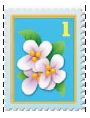i. yes
ii. no

Explanation:
The mark that is closest to the right end of the stamp is for 1 inch. So, the length of the stamp to the nearest fourth inch is 3/4 inches. Therefore the stamp is about 3/4 inch wide.

Question 2.
c.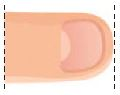i. yes
ii. no

Question 2.
d.i. yes
ii. no

### Review/Test – Page No. 618

Question 3.
Dina started swimming at 3:38 p.m. She swam until 4:15 p.m. How long did Dina swim?
________ minutes

Explanation:
STEP 1 Find 3:38 P.M. on the number line. Count on from 3:38 P.M. to 4:15 P.M. Draw marks and record the times on the number line. Then draw and label the jumps.
STEP 2 Add to find the total minutes from 3:38 P.M. to 4:15 p.M. From 3:38 P.M. to 4:15 P.M. is 37 minutes.
So, the elapsed time is 37 minutes

Question 4.
Rita’s class begins social studies at ten minutes before one in the afternoon. At what time does Rita’s class begin social studies? Circle a time that makes the sentence true.
Rita’s class begins social studies at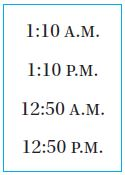__________

Explanation:
Rita’s class begins social studies at ten minutes before one in the afternoon. So, the time at which the class begins is 10 minutes subtracted from 1:00 P.M. is 12:50 P.M.

Question 5.
Select the objects with a mass greater than 1 kilogram.
Mark all that apply.
Options:
a. bicycle
b. pen
c. eraser
d. math book

Answer: a. bicycle, has a mass greater than 1 kilogram.

Explanation:
Mass is measured in grams and kilograms small quantities like paper clips, pins are measured in grams while large quantities are measured in kilograms. Therefore mass of bicycle is measured in kilograms.

Question 6.
A chicken dish needs to bake in the oven for 35 minutes. The dish needs to cool for at least 8 minutes before serving. Scott puts the chicken dish in the oven at 5:14 p.m. For numbers 6a–6d, select True or False for each statement.
a. Scott can serve the dish at 5:51 p.m.
i. True
ii. False

Question 6.
b. Scott can serve the dish at 5:58 p.m.
i. True
ii. False

Question 6.
c. Scott should take the dish out of the oven at 5:51 a.m
i. True
ii. False

Question 6.
d. Scott should take the dish out of the oven at 5:49 p.m.
i. True
ii. False

Explanation:
STEP 1 Find the ending time on the number line.
STEP 2 Count forward on the number line to add the elapsed time, 35 minutes ie. the time when the dish is kept in the oven to bake. Draw and label the jumps to show the minutes.
STEP 3 Write the times below the number line.
The jumps end at 5:49 P.M.
So, the ending time is 5:49 P.M.

### Review/Test – Page No. 619

Question 7.
Anthony’s family went out to dinner. They left at the time shown on the clock. They returned home at 6:52 p.m.Part A
How long was Anthony’s family gone?
_____ hour _____ minutes
_____ hour _____ minutes

6 hours 52 minutes

Explanation:
Anthony’s family left for dinner at 5 hours 05 minutes
Anthony’s family returned back home at 6 hours 52 minutes

Question 7.
Part B
Type below:
__________

Explanation:
STEP 1 Find 5:05 P.M. on the number line. Count on from 5:05 P.M. to 6:52 P.M. Draw marks and record the times on the number line. Then draw and label the jumps.
STEP 2 Add to find the total minutes from 5:05 P.M. to 6:52 p.M. From 5:05 P.M. to 6:52 P.M. is 107 minutes.
So, the elapsed time is 107 minutes

Question 8.
Tran checked the time on his watch after he finished his daily run.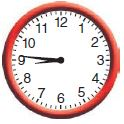Select the time that Tran finished running. Mark all that apply.
Options:
a. 14 minutes before nine
b. eight forty-six
c. quarter to nine
d. nine forty-six

Answer: a. 14 minutes before nine
b. eight forty-six

Explanation:
a. Count by fives and ones from the 12 on the clock back to where the minute hand is pointing. Write the missing counting numbers next to the clock.
b. Count on by fives and ones from the 12 on the clock to where the minute hand is pointing. Write the missing counting numbers next to the clock.
Write: 8 Hour 46 minutes

Question 9.
Cara uses a balance scale to compare mass.Circle a symbol that makes the comparison true.
The mass of the blocks img 73 the mass of the erasers.
________

Answer: mass of the blocks>mass of the erasers

Explanation:
Mass of the blocks is measured in kilograms while mass of the erasers is measured in grams.

### Review/Test – Page No. 620

Question 10.
A large bottle of water holds about 2 liters. For numbers 10a–10e, choose Yes or No to tell whether the container will hold all of the water.
a. kitchen sink
i. yes
ii. no

Explanation: A kitchen sink can hold about 2 liters. Due to the larger surface area, the kitchen sink can hold about 2 liters.

Question 10.
b. water glass
i. yes
ii. no

Question 10.
c. ice cube tray
i. yes
ii. no

Question 10.
d. large soup pot
i. yes
ii. no

Question 10.
e. lunchbox thermos
i. yes
ii. no

Explanation: Lunchbox thermos can hold about only 2 liters due to its surface area, and height.

Question 11.
Select the items that would be best measured in grams.
Mark all that apply.
Options:
a. watermelon
b. lettuce leaf
c. grape
d. onion

c. grape

Explanation: A single leaf is measured in grams.
Mass of a single grape is measured in grams.

Question 12.
Samir made a list of what he did on Tuesday. Write the letter for each activity next to the time he did it.

 A. Get out of bed. 8:05 a.m.(b) B. Walk to school. 6:25 p.m.(e) C. Eat lunch. 3:50 p.m.(d) D. Go to guitar lesson after school. 11:48 a.m.(c) E. Eat dinner at home. 6:25 a.m.(a)

### Review/Test – Page No. 621

Question 13.
Amy has 30 grams of flour. She puts 4 grams of flour in each pot of chowder that she makes. She puts 5 grams of flour in each pot of potato soup that she makes. She makes 4 pots of chowder. Does Amy have enough flour left over to make 3 pots of potato soup?
______

Explanation:
Mass of flour Amy has= 30grams
Mass of flour in each pot of chowder= 4grams
Mass of flour in each pot of potato soup= 5grams
According to the problem,
Amy makes 4 pots of chowder.
Mass of flour used to make 4 pots of chowder= 4×4= 16grams
Leftover mass of flour=30-16=14 grams
Mass of flour used to make 3 pots of potato soup=5×3=15 grams
But, Amy only has 14 grams. So, we can say that Amy doesn’t have enough flour leftover to make 3 pots of potato soup.

Question 14.
Use an inch ruler to measure.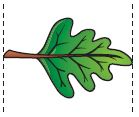Part A
What is the length of the leaf to the nearest fourth inch?
Type below:
__________

Explanation:
Line up the left end of the leaf with the zero mark on the ruler.
The right end of the leaf is between the fourth-inch marks for 2 and 3.
The mark that is closest to the right end of the leaf is for 1/4 inches.
So, the length of the leaf to the nearest fourth inch is 2 1/4 inches.

Question 14.
Part B
Explain what happens if you line up the left side of the object with the 1 on the ruler.
Type below:
__________

Explanation:
Line up the left end of the object with the one mark on the ruler.
The right end of the paper clip is between the fourth-inch marks for 2 and 3.
The mark that is closest to the right end of the paper clip is for 1/4 inches.
So, the length of the paper clip to the nearest fourth inch is 1 1/4 inches.

Question 15.
Mrs. Park takes the 9:38 a.m. train to the city. The trip takes 3 hours and 20 minutes. What time does Mrs. Park arrive in the city?
______ : _______ _______

Explanation:
STEP 1 Find the ending time on the clock, the time at which Mrs. Park arrive in the city.
STEP 2 Count forward by hours and then by fives for the elapsed time of 3 hours 20 minutes. Write the missing counting numbers next to the clock.
So, the ending time is 12:58 P.M.

Question 16.
Hector buys two bags of gravel for his driveway. He buys a total of 35 kilograms of gravel. Select the bags he buys.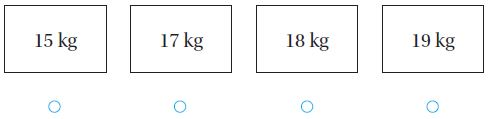Answer: 17 kg and 18 kg

Explanation:
Hector buys two bags of gravel for his delivery.
Hector buys a total of 35 kilograms of gravel.
According to the problem,
The bags selected by Hector are 17 kg and 18 kg because 17+18=35 kg

### Review/Test – Page No. 622

Question 17.
Ashley measures the shells she collects. She records the measurements in a chart.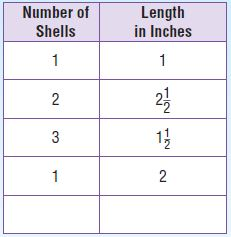Part A
Ashley found a razor clam shell this long. Use an inch ruler to measure. Record the measurement in the chart.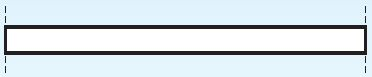_____ $$\frac{□}{□}$$ inches

Question 17.
Part B
Complete the line plot to show the data in the chart. How many shells are longer than 2 inches? Tell how you know.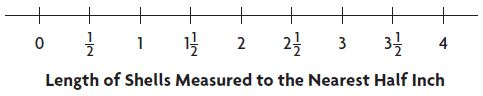_______ shells

Explanation:
Calculate the number of shells which are longer than 2 inches.
By observing the number line we find 2. From 2 draw curves to the next values in such a way that the other values are greater than 2.
We need to draw the curves till 4.
Count the curves drawn from 2 to 4 the number of curves=number of shells (which are measured to the nearest half-inch)=4

Question 18.
Lucy fills a bathroom sink with water. Is the amount of water more than 1 liter, about 1 liter, or less than 1 liter?
Explain how you know.
________